JEE  >  True/False: Trigonometric Functions & Equations | JEE Advanced

# True/False: Trigonometric Functions & Equations | JEE Advanced - Notes | Study Maths 35 Years JEE Main & Advanced Past year Papers - JEE

 1 Crore+ students have signed up on EduRev. Have you?

Q.1. If  tan A = (1- cos B)/sinB , then tan 2A = tan B. (1983 - 1 Mark)

Ans. T

Sol.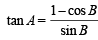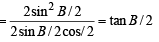Hence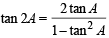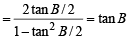∴ Statement is true.

Q.2. There exists a value of  θ between 0 and 2π that satisfies the equation sin4θ - 2 sin2θ - 1 = 0 .   (1984 - 1 Mark)

Ans. F

Sol.  Given equation is sin4θ- 2 sin2θ - 1=0 Here,

D = 4 + 4 = 8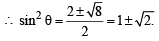But sin2θ can not be –ve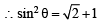But as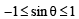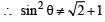Thus there is no value of θ which satisfy the given equation.

∴ Statement is false.

The document True/False: Trigonometric Functions & Equations | JEE Advanced - Notes | Study Maths 35 Years JEE Main & Advanced Past year Papers - JEE is a part of the JEE Course Maths 35 Years JEE Main & Advanced Past year Papers.
All you need of JEE at this link: JEE

## Maths 35 Years JEE Main & Advanced Past year Papers

132 docs|70 tests
 Use Code STAYHOME200 and get INR 200 additional OFF

## Maths 35 Years JEE Main & Advanced Past year Papers

132 docs|70 tests

Track your progress, build streaks, highlight & save important lessons and more!

,

,

,

,

,

,

,

,

,

,

,

,

,

,

,

,

,

,

,

,

,

;